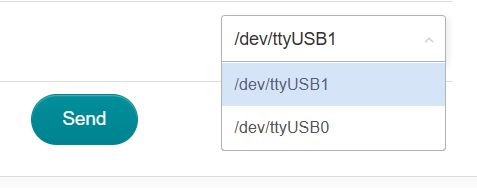# X750 need help, 4g modem no working

hello , I have a 4g router gl x750 , and I made a mistake， I send a wrong AT commands “AT+QCFG=“usbnet”,3” to 4g modem ,and then the 4g module does not work any more, and a few options are missing in the device setting, there is an extra usb0 in the hotspot sharing.I tried to send the correct command, all failed.I tried reset router and reset modem button, also restore firmware, reinstall firmware, still the same. Ho
w can I really reset the 4g modem and restore the previous state. thanks

To reset the Quectel EC25 module to its factory state, you can use the AT command “AT+QRST=1”. This command will perform a “hard reset” and restore the module’s default settings.

thank you, but problem is right now only have /dev/ttyUSB0 and /dev/ttyUSB1 I can choose, No matter which device I choose to send the command, it all fails. Is there any other way to send the command?This is indeed an issue, I’ll ask my colleague for help.

I managed to revert back to modem mode. The most important thing is to use an operating system with a kernel version above 5.10. OpenWrt 22.03 will work. Please upgrade to the upstream OpenWrt for this task.

``````cat /sys/kernel/debug/usb/devices
``````
``````T:  Bus=01 Lev=01 Prnt=01 Port=00 Cnt=01 Dev#=  2 Spd=480  MxCh= 0
D:  Ver= 2.00 Cls=ef(misc ) Sub=02 Prot=01 MxPS=64 #Cfgs=  1
P:  Vendor=2c7c ProdID=0125 Rev= 3.18
S:  Manufacturer=Android
S:  Product=Android
C:* #Ifs= 6 Cfg#= 1 Atr=a0 MxPwr=500mA
A:  FirstIf#= 0 IfCount= 2 Cls=e0(wlcon) Sub=01 Prot=03
I:* If#= 0 Alt= 0 #EPs= 1 Cls=e0(wlcon) Sub=01 Prot=03 Driver=rndis_host
E:  Ad=82(I) Atr=03(Int.) MxPS=   8 Ivl=32ms
I:* If#= 1 Alt= 0 #EPs= 2 Cls=0a(data ) Sub=00 Prot=00 Driver=rndis_host
E:  Ad=81(I) Atr=02(Bulk) MxPS= 512 Ivl=0ms
E:  Ad=01(O) Atr=02(Bulk) MxPS= 512 Ivl=0ms
I:* If#= 2 Alt= 0 #EPs= 2 Cls=ff(vend.) Sub=ff Prot=ff Driver=option
E:  Ad=83(I) Atr=02(Bulk) MxPS= 512 Ivl=0ms
E:  Ad=02(O) Atr=02(Bulk) MxPS= 512 Ivl=0ms
I:* If#= 3 Alt= 0 #EPs= 3 Cls=ff(vend.) Sub=00 Prot=00 Driver=option
E:  Ad=85(I) Atr=03(Int.) MxPS=  10 Ivl=32ms
E:  Ad=84(I) Atr=02(Bulk) MxPS= 512 Ivl=0ms
E:  Ad=03(O) Atr=02(Bulk) MxPS= 512 Ivl=0ms
I:* If#= 4 Alt= 0 #EPs= 3 Cls=ff(vend.) Sub=00 Prot=00 Driver=option
E:  Ad=87(I) Atr=03(Int.) MxPS=  10 Ivl=32ms
E:  Ad=86(I) Atr=02(Bulk) MxPS= 512 Ivl=0ms
E:  Ad=04(O) Atr=02(Bulk) MxPS= 512 Ivl=0ms
I:* If#= 5 Alt= 0 #EPs= 3 Cls=ff(vend.) Sub=00 Prot=00 Driver=option
E:  Ad=89(I) Atr=03(Int.) MxPS=  10 Ivl=32ms
E:  Ad=88(I) Atr=02(Bulk) MxPS= 512 Ivl=0ms
E:  Ad=05(O) Atr=02(Bulk) MxPS= 512 Ivl=0ms
``````

The AT port is `if#=4` when it’s in usbnet mode. That port must be bound with the `option` driver.

To revert use command:

``````echo 'AT+QCFG="usbnet",0' >/dev/ttyUSB2
echo 'AT+CFUN=1,1' >/dev/ttyUSB2
``````

Then wait the modem reboot itself.

3 Likes